# Modular Form ii

This is a continuation of ideas from before, except we now embrace color. But we don't use the typical colors.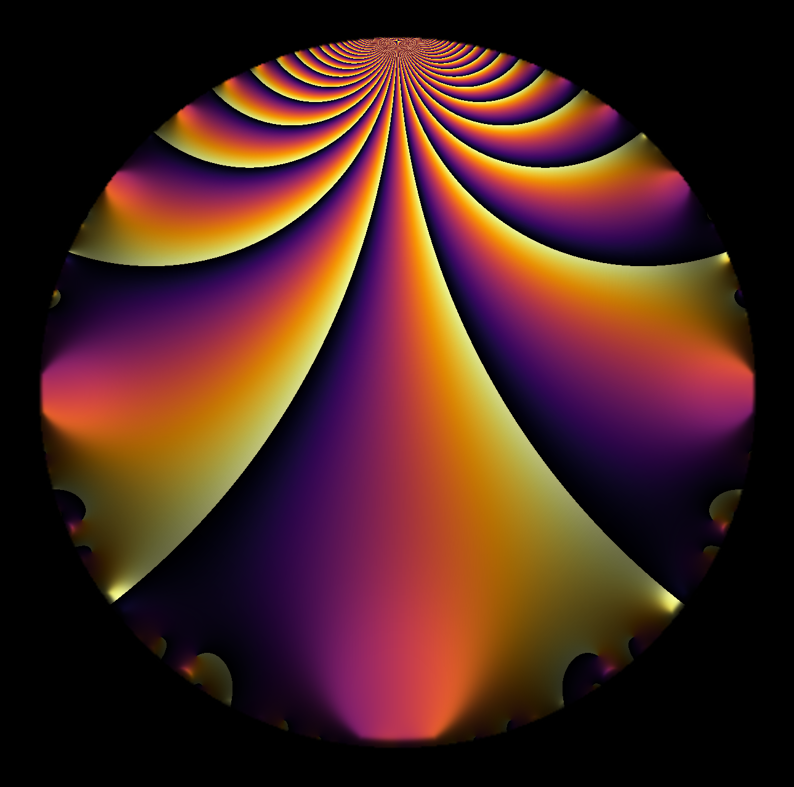A modular form impersonating a stage curtain. DLD Oct 2020.

A complex-valued function $$f: \mathbb{C} \longrightarrow \mathbb{C}$$ naturally has a four dimensional graph. We represent this in two dimensions here by representing each point $$z$$ in the codomain by $$z = r e^{i \theta}$$, for a magnitude $$r$$ and argument $$\theta$$. Here, we choose colors based on $$\theta$$, and we encode the magnitude in a variety of ways.

Sometimes we use contours in indicate the magnitude.

Or we use darkness or lightness to indicate the magnitude.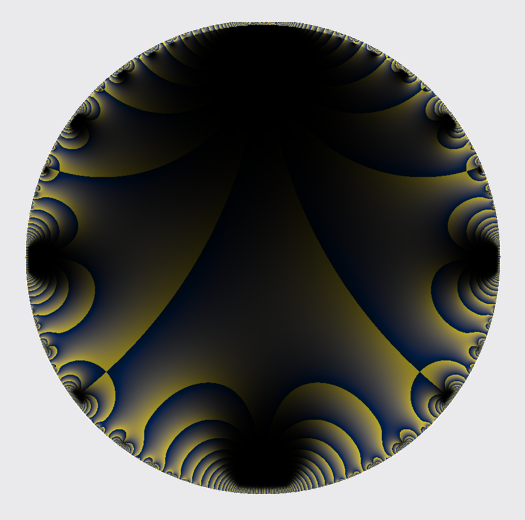$\Delta(z)$ in yellow, blue, and black. DLD Oct 2020.

Choosing small windows, various forms of self-similarity make themselves obvious. These images have a flow much like the surface of a stream. Colors swirl in eddies and whirlpools.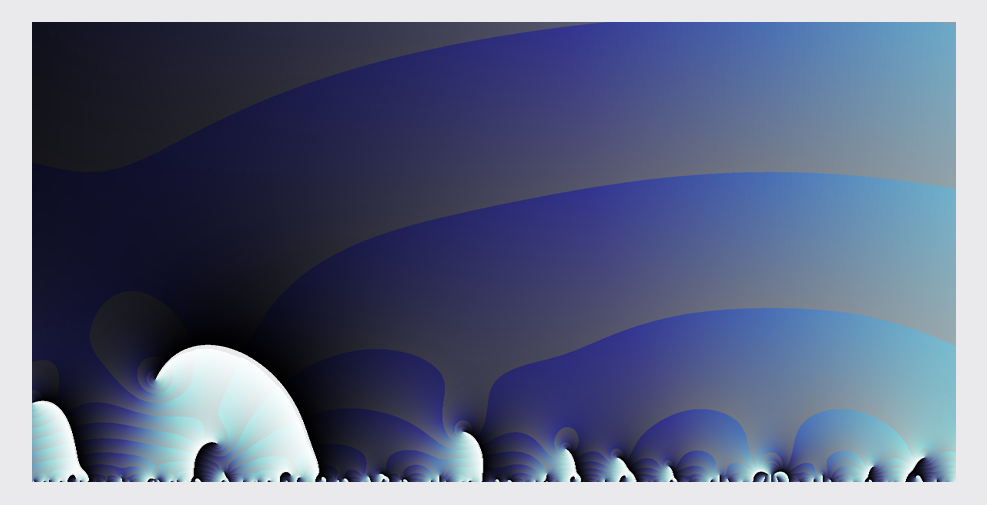Wind over mountain peaks. DLD Oct 2020.

Small differences in color can have strong associative effect. The following image is essentially the same as Features in the mist i, from before, but with colors inverted and on a grey paper background.

This is a modular form, but it looks like a speckled marble, perhaps a solar map.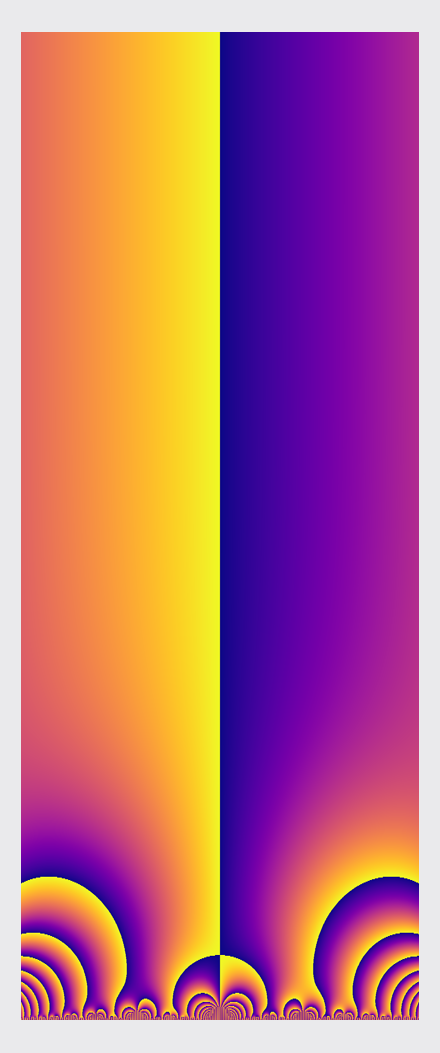Untitled modular form banner. DLD Oct 2020.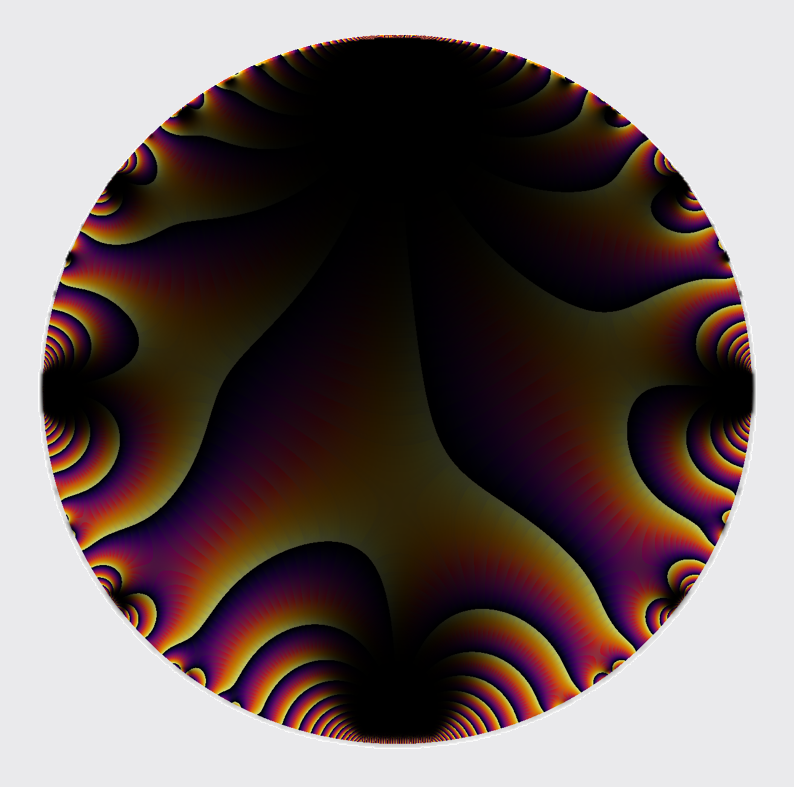Untitled modular form medallion. DLD Oct 2020.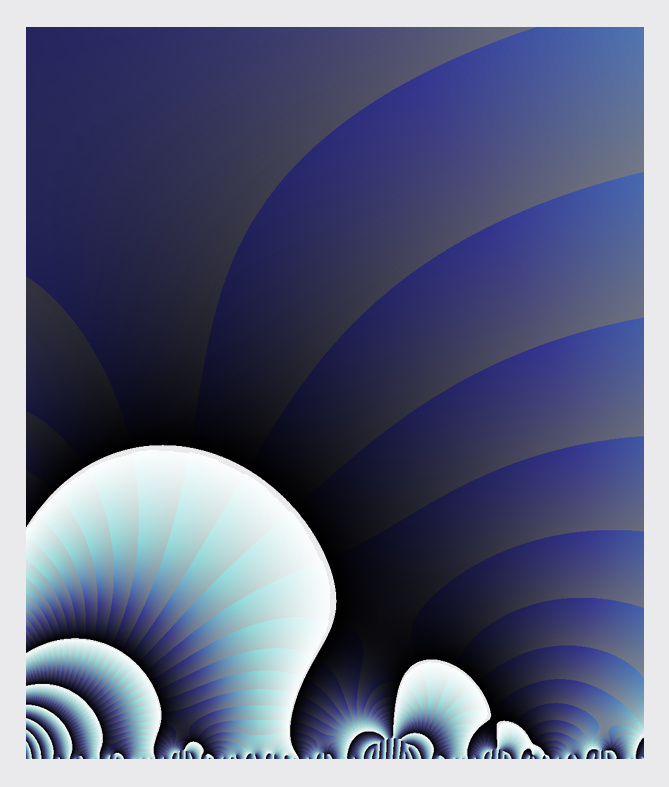Untitled modular form detail. DLD Oct 2020.